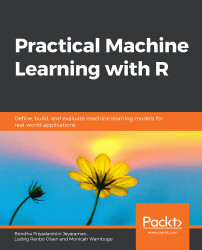•#### Practical Machine Learning with R#### Overview of this book

With huge amounts of data being generated every moment, businesses need applications that apply complex mathematical calculations to data repeatedly and at speed. With machine learning techniques and R, you can easily develop these kinds of applications in an efficient way. Practical Machine Learning with R begins by helping you grasp the basics of machine learning methods, while also highlighting how and why they work. You will understand how to get these algorithms to work in practice, rather than focusing on mathematical derivations. As you progress from one chapter to another, you will gain hands-on experience of building a machine learning solution in R. Next, using R packages such as rpart, random forest, and multiple imputation by chained equations (MICE), you will learn to implement algorithms including neural net classifier, decision trees, and linear and non-linear regression. As you progress through the book, you’ll delve into various machine learning techniques for both supervised and unsupervised learning approaches. In addition to this, you’ll gain insights into partitioning the datasets and mechanisms to evaluate the results from each model and be able to compare them. By the end of this book, you will have gained expertise in solving your business problems, starting by forming a good problem statement, selecting the most appropriate model to solve your problem, and then ensuring that you do not overtrain it.Free Chapter
An Introduction to Machine LearningData Cleaning and Pre-processingFeature EngineeringIntroduction to neuralnet and Evaluation MethodsLinear and Logistic Regression ModelsUnsupervised LearningAppendix## Summary

In this chapter, we have learned about the different types of features that are generated to train a model. We have derived domain-specific features and datatype-specific features. Also, we explored an automated technique for generating text features. The feature engineering process is essential for obtaining the best model performance. We delved into two variable transformation techniques and learned about techniques to identify redundant features and handle them in a dataset.

We have learned about forward and backward feature selection approaches and have performed correlation analysis through detailed examples. We implemented the calculation of p-values in R and looked at its significance to the process of the selection of features. Recursive feature elimination is another way that we saw to find the best combination of features for a model. We delved into a dimensionality reduction approach, known as PCA, that drastically reduces the number of features needed, as it calculates...